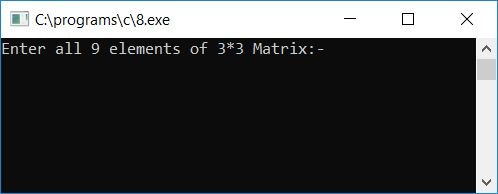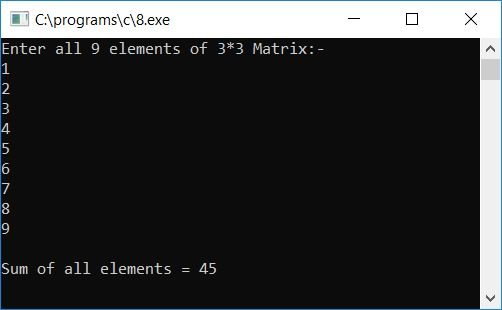# C program to find the sum of all the elements of a matrix

In this article, you will learn and get code for finding the sum of all elements of a given matrix.

The question is: Write a C program that takes nine elements of a 3*3 matrix as input from the user (at run-time) and calculates the sum of all the matrix elements. The answer to this question is:

```#include<stdio.h>
#include<conio.h>
int main()
{
int mat, i, j, sum;
sum = 0;
printf("Enter all 9 elements of 3*3 Matrix:-\n");
for(i=0; i<3; i++)
{
for(j=0; j<3; j++)
{
scanf("%d", &mat[i][j]);
sum = sum + mat[i][j];
}
}
printf("\nSum of all elements = %d", sum);
getch();
return 0;
}```

The program was written in the Code::Blocks IDE; therefore, after a successful build and run, you will get the output as shown in the sample given here:Enter all 9 elements and press the enter key. Here is the second screenshot of the sample run:#### Program Explained

1. Take all 9 elements as input and initialize the matrix one by one.
2. The 3*3 matrix means it has 3 rows and 3 columns.
3. We have to use two for loops; the outer one is for rows, and the inner one is for columns.
4. In this manner
• The first element is set to mat.
• The second element is set to mat.
• The third element is set to mat.
• Similarly, the fourth, fifth, sixth, seventh, eighth, and ninth elements are initialized to mat, mat, mat, mat, mat, and mat.
5. At the time of scanning every element, the summation statement is applied.
6. Finally, print out the value of the sum variable. Never forget to set the sum variable to 0 before attempting to compute the sum of all matrix elements.

C Quiz

« Previous Program Next Program »